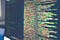xorpd has some riddle-like pieces of assembly code here. In this post, I’ll analyze riddles 0x12, 0x0d and 0x0e which has some things in common. Let’s get to it!Photo by Markus Spiske on Unsplash

# xorpd riddle 0x12

Here’s the code:

`mov      rcx,rdxand      rdx,raxor       rax,rcxadd      rax,rdx`

Let’s go step by step:

`mov      rcx,rdx  ; rcx =  xand      rdx,rax  ; rdx =  x && yor       rax,rcx  ; rax =  y || xadd      rax,rdx  ; rax = (y || x) + (x && y)`

The interesting fact about this expression is that is equivalent to an or. In order to show you this, I’ll conduct some tests. Imaging every unknown value to be a single bit, plausible cases are:

`| X | Y |---------| 0 | 0 || 0 | 1 || 1 | 0 || 1 | 1 |`

Taking apart the expression is easy to notice that:

• The last part (x && y) will only be true if both variables are true
• The first part (x || y) will only be false if both variables are false

We can clearly see that these observations overlap with some of the + operator (add mnemonic), in fact, if we compute the truth table for the whole expression (named exp) and add we can observe they are equivalent:

`| X | Y | =>  | exp | add |---------    -------------| 0 | 0 |  => |   0 |   0|| 0 | 1 |  => |   1 |   1|| 1 | 0 |  => |   1 |   1|| 1 | 1 |  => |  10 |  10|`

# xorpd riddle 0x0d

This time the code looks like this:

`mov      rdx,rbxxor      rbx,rcxand      rbx,raxand      rdx,raxand      rax,rcxxor      rax,rdxcmp      rax,rbx`

What’s going on here? We have 3 unknown values coming from registers rbx, rcx and rax. Let’s call them X, Y, Z. If we replace the register for variables in the code snippet we get the following:

`mov      rdx,rbx  ; rdx = Xxor      rbx,rcx  ; rbx = X ^ Yand      rbx,rax  ; rbx = Z & (X ^ Y)and      rdx,rax  ; rdx = Z & Xand      rax,rcx  ; rax = Z & Yxor      rax,rdx  ; rax = (Z & Y) ^ (Z & X)cmp      rax,rbx  ; ((Z & Y) ^ (Z & X)) - (Z & (X ^ Y))`

Having followed through this stream of xor and and ops we reach the following simplified comparison at the end:

`cmp (Z & (X ^ Y)), ((Z & Y) ^ (X & Z))`

In the end, both expressions will have the same value, regardless of the initial values of the registers. This happens because of the distributive property of and over xor, which derives from the De Morgan’s laws.

# xorpd riddle 0x0e

Let’s analyze the third one:

`mov      rcx,raxand      rcx,rbxnot      rcxnot      raxnot      rbxor       rax,rbxcmp      rax,rcx`

Replacing the unknown values with variables X and Y give us some insight:

`mov      rcx,rax  ; rcx = Xand      rcx,rbx  ; rcx = X & Ynot      rcx      ; rcx = !(X && Y)not      rax      ; rax = !Xnot      rbx      ; rbx = !Yor       rax,rbx  ; rax = !X || !Y cmp      rax,rcx  ; cmp !X || !Y, !(X && Y)`

We can see that, similar to the previous riddle, the last statement will always be true as it corresponds with the following De Morgan’s Law:

`!X || !Y is equivalent to !(X && Y)`

So, regardless of the initial values for rax and rbx, rax and rcx will have the same value at the end.

Written by

## syscall59

#### Shellcode for the masses

Welcome to a place where words matter. On Medium, smart voices and original ideas take center stage - with no ads in sight. Watch
Follow all the topics you care about, and we’ll deliver the best stories for you to your homepage and inbox. Explore
Get unlimited access to the best stories on Medium — and support writers while you’re at it. Just \$5/month. Upgrade